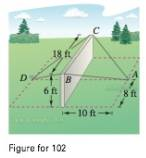Chapter 11.2, Problem 104E

Chapter
Section
Textbook Problem

# Construction A precast concrete wall is temporarily kept in its vertical position by ropes (see figure). Find the total force exerted on the pin at position A The tensions in AB and AC are 420 pounds and 650 pounds, respectively.To determine
The total force exerted at on the pin at point A with the given data..

Explanation

Given: The tension on AB=420 pounds, AC=650 pounds is given.

Explanation: It can be seen from the figure, consider Coordinates of points A, B, C, D are (0, 10, 0), (8,-10, 6), and (-10, 0, 6) respectively.

The AB is,

AB=80,010,60=8,10,6

Therefore, T1 is,

T1=c18,10,6

Now,

T1=c182+(10)2+62=c1100+100=c1102c1102=420c1422=212

Finally T1 is,

T1=2128,10,6

The vector AC is,

AC=100,010,60=10,10,6

So,

T2=c210,10,6T2=c2(10)2+(10)2+62=c2100+100+36=c2236

### Still sussing out bartleby?

Check out a sample textbook solution.

See a sample solution

#### The Solution to Your Study Problems

Bartleby provides explanations to thousands of textbook problems written by our experts, many with advanced degrees!

Get Started

#### In Problems 35-42, simplify each complex fraction. 38.

Mathematical Applications for the Management, Life, and Social Sciences

#### The quadratic approximation for at a = 1 is:

Study Guide for Stewart's Multivariable Calculus, 8th

#### The slope of the tangent line to y = x3 at x = 2 is: 18 12 6 0

Study Guide for Stewart's Single Variable Calculus: Early Transcendentals, 8th